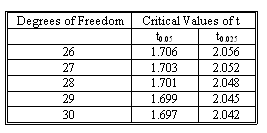### CFA Practice Question

There are 364 practice questions for this topic.

### CFA Practice Question

You have 3 independent variables and 30 observations. The t-statistic for one of the independent variables is 2.013. What is the critical value for Student's t distribution? Is the variable significant at the 5% confidence level?Correct Answer: The critical value is 2.056 and the variable is not significant.

The degrees of freedom is 30 - 3 - 1 = 26. Because the Student's t distribution is for the area in the upper tail, the critical value is based on 2.5%. Therefore, the critical value for 26 degrees of freedom is 2.056. Because the t-statistic is only 2.013, it is not significant.